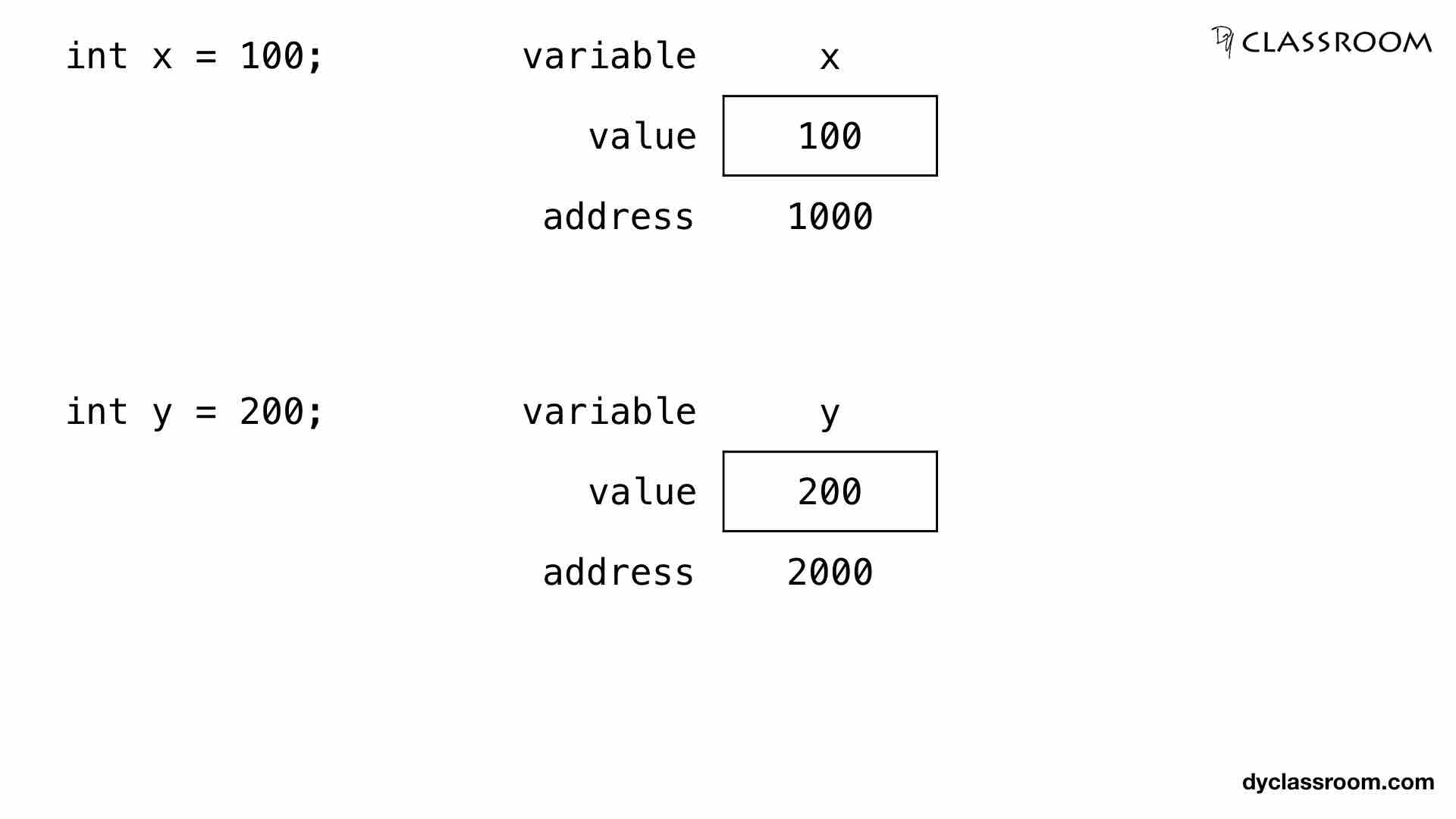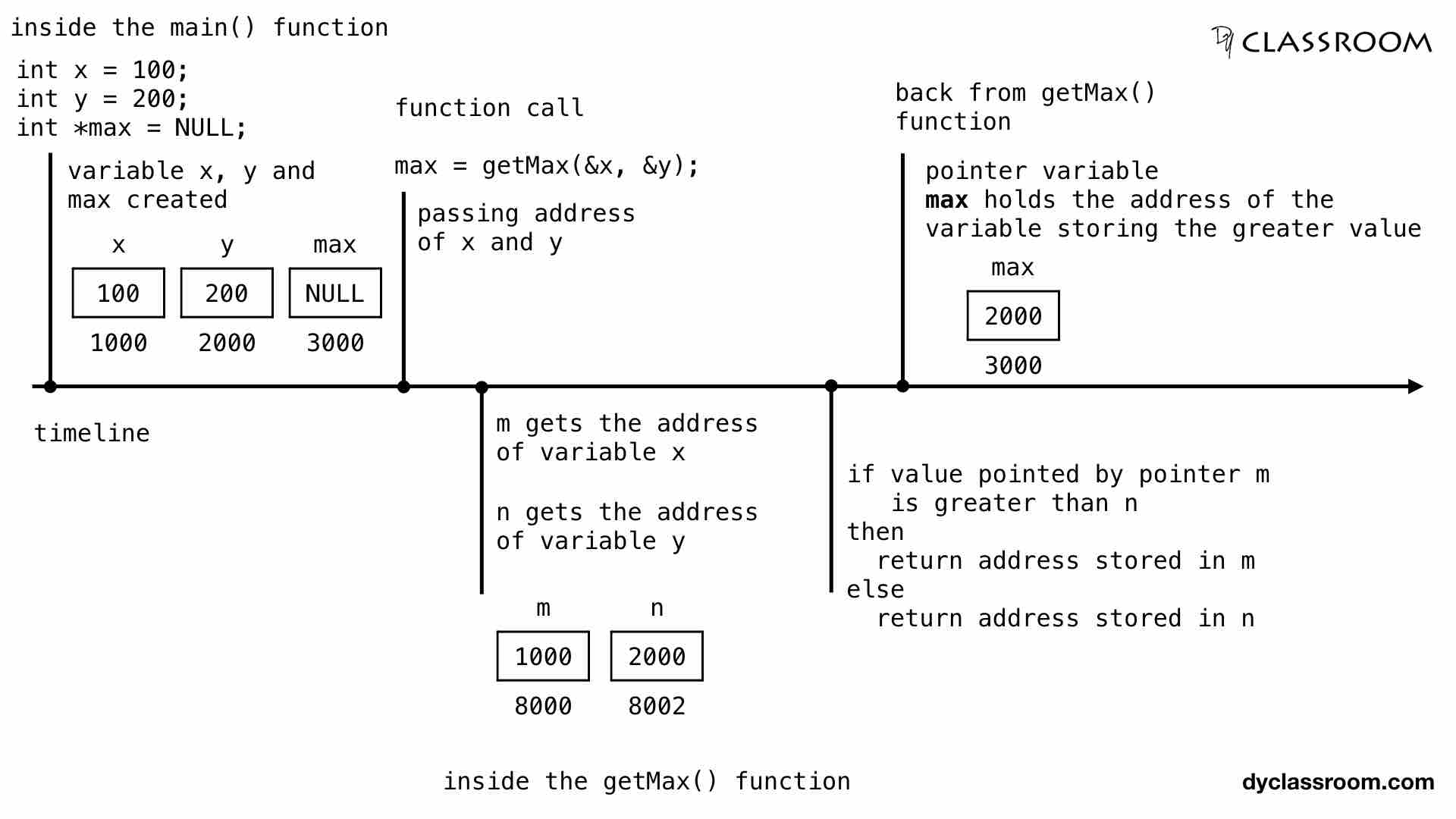# C - Function returning pointer

C ProgrammingIn this tutorial we will learn to return pointer from function in C programming language.

In the previous tutorial we learned how to create functions that will accept pointers as argument.

Now, let us go ahead and create a function that will return pointer.

## Declaration of function that returns pointer

Following is the function declaration syntax that will return pointer.

``````returnType *functionName(param list);
``````

Where, `returnType` is the type of the pointer that will be returned by the function `functionName`.

And `param list` is the list of parameters of the function which is optional.

In the following example we are declaring a function by the name `getMax` that takes two integer pointer variable as parameter and returns an integer pointer.

``````int *getMax(int *, int *);
``````

## Function that returns pointer

In the following example the `getMax()` function returns an integer pointer i.e., address of a variable that holds the greater value.

``````#include <stdio.h>

// function declaration
int *getMax(int *, int *);

int main(void) {

// integer variables
int x = 100;
int y = 200;

// pointer variable
int *max = NULL;

/**
* get the variable address that holds the greater value
* for this we are passing the address of x and y
* to the function getMax()
*/
max = getMax(&x, &y);

// print the greater value
printf("Max value: %d\n", *max);

return 0;
}

// function definition
int *getMax(int *m, int *n) {

/**
* if the value pointed by pointer m is greater than n
* then, return the address stored in the pointer variable m
*/
if (*m > *n) {
return m;
}
/**
* else return the address stored in the pointer variable n
*/
else {
return n;
}

}
``````

Output:

``````Max value: 200
``````

We can represent the two variables `x` and `y` in memory as follows.So, in the above image we can see that variable `x` is stored in the memory location 1000 and variable `y` is stored in the memory location 2000.

Then we are creating an integer pointer variable `max` which will hold the address of the variable that has the greater value. At this moment pointer variable `max` is stored at the memory location 3000 and it stores NULL value.Next we are calling the `getMax()` function and passing the address of variable `x` and `y`.

In the `getMax()` function the parameter `m` gets the address of variable `x` and parameter `n` gets the address of variable `y`.

So, integer pointer variable m holds the address of the variable x i.e., 1000 and is allocated the memory location 8000.

Similary, integer pointer variable n is allocated the memory location 8002 and it holds the address of the variable y i.e., 2000.

Now, we are checking is `*m > *n` i.e., is value pointed by the pointer variable m greater than the value pointed by the pointer variable n.

Value pointed by pointer variable m is 100 and value pointed by pointer variable n is 200. So, the if-block is ignored and the else-block is executed which returns the address stored in the pointer variable n i.e., 2000 which is the address of the variable y.

So, address of variable y is returned from `getMax()` function and it is stored in the integer pointer variable `max`. So, max variable is now holding the address 2000.

Finally, we are printing the value stored in the address 2000 via pointer variable max. This will give us the greater value i.e., 200.